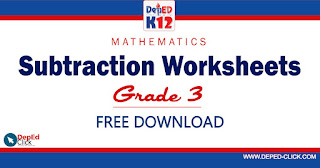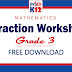Mathematics are the result of mysterious powers which no one understands, and which the unconscious recognition of beauty must play an important part. Out of an infinity of designs a mathematician chooses one pattern for beauty’s sake and pulls it down to earth.
— Marston MorseSUBTRACTION WORKSHEETS for Grade 3 Subtraction Worksheet 1 Subtraction Worksheet 2 Subtraction Worksheet 3 Subtraction Worksheet 4 Subtraction Worksheet 5 Subtraction Worksheet 6

SUBTRACTION WORKSHEETS (Grade 3) Free DownloadReviewed by DepEd Click on May 09, 2020 Rating: 5